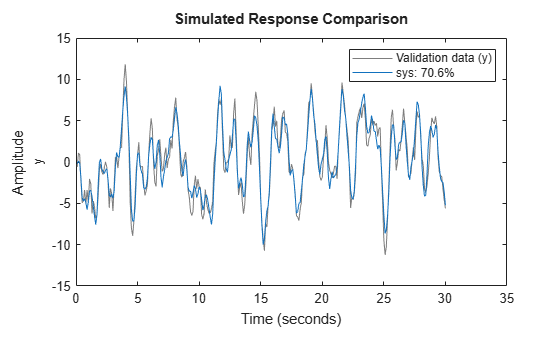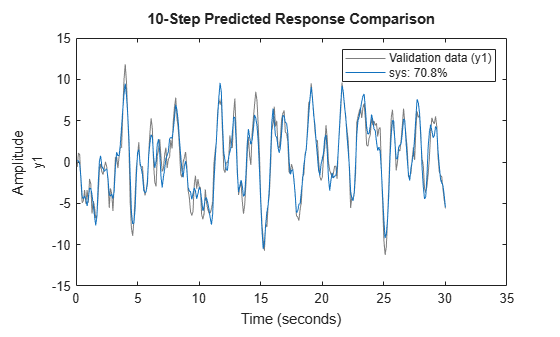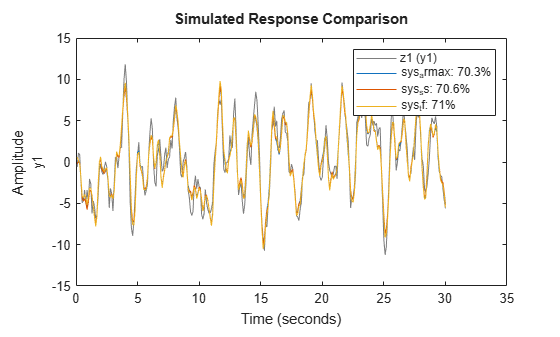Documentation

compare

Compare identified model output and measured output

Description

Plot Results

example

compare(data,sys) simulates the response of a dynamic system model, and superimposes that response over plotted measurement data. The plot also displays the normalized root mean square (NRMSE) measure of the goodness of the fit between simulated response and measurement data. Use this function when you want to evaluate a set of candidate models identified from the same measurement data, or when you want to validate a model you have selected. You can use compare with time-domain or frequency-domain models and data.

example

compare(data,sys,kstep) also predicts the response of sys, using a prediction horizon specified by kstep. Prediction uses output measurements as well as input measurements to project a future response. kstep represents the number of time samples between the timepoint of each output measurement and the timepoint of the resulting predicted response. For more information on prediction, see Simulate and Predict Identified Model Output.

compare(data,sys,LineSpec,kstep) also specifies the line type, marker symbol, and color for the model response.

example

compare(data,sys1,...,sysN,kstep) compares the responses of multiple dynamic systems on the same axes. compare automatically chooses the line specifications.

example

compare(data,sys1,LineSpec1,...,sysN,LineSpecN,kstep) also compares the responses of multiple systems on the same axes using the line type, marker symbol, and color specified for each system.

example

compare(___,opt) configures the comparison using an option set. Options include initial condition handling, data offsets, and data selection. You can use this syntax with any of the previous input-argument combinations.

Return Results

[y,fit,x0] = compare(___) returns the model response, y, goodness of fit value, fit, and the initial states, x0. No plot is generated. You can use this syntax with any of the previous input-argument combinations. However, line specifications are ignored, because there is no plotting.

Examples

collapse all

Identify a linear model and visualize the simulated model response with the data from which it was generated.

Load input/output measurements z1, and identify a third-order state-space model sys.

sys = ssest(z1,3);

sys is a continuous-time identified state-space (idss) model.

Use compare to simulate the sys response and plot it alongside the data z1.

figure
compare(z1,sys)The plot illustrates the differences between the model response and the original data. The percentage shown in the legend is the NRMSE fitness value. It represents how close the predicted model output is to the data.

To change display options in the plot, right-click the plot to access the context menu. For example:

• To plot the error between the predicted output and measured output, select Error Plot.

• To view the confidence region for the simulated response, select Characteristics -> ConfidenceRegion.

• To specify number of standard deviations to plot, double-click the plot and open the Property Editor dialog box. In the Options tab, specify the number of standard deviations in Confidence Region for Identified Models. The default value is 1 standard deviation.

Identify a linear model and visualize the predicted model response with the data from which it was computed.

Identify a third-order state-space model using the input/output measurements in z1.

sys = ssest(z1,3);

sys is a continuous-time identified state-space (idss) model.

Now use compare to plot the predicted response. Prediction differs from simulation in that it uses both measured input and measured output when computing the system response. The prediction horizon defines how far in the future to predict, relative to your current measured output point. For this example, set the prediction horizon kstep to 10 steps, and use compare to plot the predicted response against the original measurement data.

kstep = 10;
compare(z1,sys,kstep)In this plot, each sys data point represents the predicted output associated with output measurement data that was taken at least 10 steps earlier. For instance, the point at t = 15s is based on output measurements taken at or prior to t = 5s. The calculation of this t = 15s sys data point also uses input measurements up to t = 15s, just as a simulation would.

The plot illustrates the differences between the model response and the original data. The percentage shown in the legend is the NRMSE fitness value. It represents how closely the predicted model output matches the data.

To change display and simulation options in the plot, right-click the plot to access the context menu. For example, to plot the error between the predicted output and measured output, select Error Plot from the context menu. To change the prediction horizon value, or to toggle between simulation and prediction, select Prediction Horizon from the context menu.

Identify several model types for the same data, and compare the results to see which best fits the data.

Load the data, which contains iddata object z1 with single input and output.

From z1, identify a model for each of the following linear forms:

• ARMAX (idpoly) of orders 2, 3, and 1, with dead time of 0

• State space (idss) with three states

• Transfer function (idtf) with three poles

sys_armax = armax(z1,[2 3 1 0]);
sys_ss = ssest(z1,3);
sys_tf = tfest(z1,3);

Using compare, plot the simulated responses for the three models with z1.

compare(z1,sys_armax,sys_ss,sys_tf)For this set of data, along with the default settings for all the models, the transfer-function form has the best NRMSE fit. However, the fits for all models are within about 1% of each other.

You can interactively control which model responses are displayed in the plot by right-clicking on the plot and hovering over Systems.

Compare the outputs of multiple estimated models of differing types to measured frequency-domain data.

For this example, estimate a process model and an output-error polynomial from frequency response data.

load demofr  % frequency response data
zfr = AMP.*exp(1i*PHA*pi/180);
Ts = 0.1;
data = idfrd(zfr,W,Ts);
sys1 = procest(data,'P2UDZ');
sys2 = oe(data,[2 2 1]);

sys1, an idproc model, is a continuous-time process model. sys2, an idpoly model, is a discrete-time output-error model.

Compare the frequency response of the estimated models to data.

compare(data,sys1,'g',sys2,'r');The two models have NRMSE fit values that are nearly equal with respect to the data from which they were calculated.

Modify default behavior when you compare an estimated model to measured data.

Estimate a transfer function for measured data.

sys = tfest(z1,3);

sys is a continuous-time identified transfer function (idtf) model.

Suppose you want your initial conditions to be zero. The default for compare is to estimate initial conditions from the data.

Create an option set to specify the initial condition handling. To use zero for initial conditions, specify 'z' for the 'InitialCondition' option.

opt = compareOptions('InitialCondition','z');

Compare the estimated transfer function model output to the measured data using the comparison option set.

compare(z1,sys,opt)Input Arguments

collapse all

Validation data, specified as an iddata, idfrd, or frd object.

If sys is:

• An iddata object, then data must be an iddata object with matching domain, number of experiments and time or frequency vectors

• A frequency-response data (FRD) model (defined as either idfrd or frd), then data must also be FRD

• A parametric model (such as idss), then data can be iddata or FRD

data can represent either time-domain or frequency-domain data when comparing with linear models. data must be time-domain data when comparing with a nonlinear model.

For examples, see:

.

Identified model, specified as a dynamic system model, an iddata object, or a model array.

When the time or frequency units of data do not match the units of sys, compare rescales sys to match the units of data.

Prediction horizon, specified as one of the following:

• Inf — Compare simulated response of sys to data.

• Positive finite integer — Compare predicted response of sys to data, where each predicted response point is based not only on measured input data up to that timepoint, but also on measured output data up to kstep timepoints earlier.

compare ignores kstep when sys is an iddata object, an FRD model, or a dynamic system with no noise component. compare also ignores kstep when using frequency response validation data.

If you specify kstep that is greater than the number of data samples, compare sets kstep to Inf and provides a warning message.

For more information on simulation and prediction, see Simulate and Predict Identified Model Output.

For an example, see Compare Predicted Response of Identified Time-Domain Model to Measured Data.

Line style, marker, and color of both the line and marker, specified as a character vector, such as 'b' or 'b+:'.

For more information about configuring LineSpec, see the Linespec input argument of plot. For an example, see Compare Multiple Estimated Models to Measured Frequency-Domain Data.

Comparison options, specified as an option set you create using compareOptions.

Available options include:

• Handling of initial conditions

• Sample range for computing fit numbers

• Data offsets

• Output weighting

For examples, see:

Output Arguments

collapse all

Model response, returned as an iddata object, an idfrd object, a cell array, or an array. The output depends on the models and data you provide, as follows:

• For a single model and single-experiment data set, y is an iddata object or idfrd object

• For multimodel comparisons, y is a cell array with one iddata or idfrd object entry for each input model

• For multiexperiment data, y is a cell array with one entry for each experiment

• For multimodel comparisons using multiexperiment data, y is an Nsys-by-Nexp cell array, where Nsys is the number of models, and Nexp is the number of experiments

• If sys is a model array, y is an array with an element corresponding to each model in sys and experiment in data. For more information on model arrays, see stack

If kstep is not specified or is Inf, then compare returns the simulated response in y.

Otherwise, compare returns the predicted response. Measured output values in data up to time tn-kstep are used to predict the output of sys at time tn. For more information on simulation and prediction, see Simulate and Predict Identified Model Output.

The compare response computation requires specification of initial condition handling. By default, compare estimates the initial conditions to maximize the fit to data. See compareOptions for more information on how compare determines the initial conditions to use.

NRMSE fitness value indicator of how well the simulated or predicted model response matches the measurement data, returned as a vector, a matrix, or a cell array. The output depends on the models and data you provide, as follows:

• If data is an iddata object, fit is a vector of length Ny, where Ny is the number of outputs

• If data is an FRD model, fit is an Ny-by-Nu matrix, where Nu is the number of inputs in data

• For a single model and single-experiment data set, fit is a vector or matrix

• For multimodel comparisons, fit is a cell array with one entry for each input model

• For multiexperiment data, fit is a cell array with one entry for each experiment

• For multimodel comparisons using multiexperiment data, fit is an Nsys-by-Nexp cell array, where Nsys is the number of models, and Nexp is the number of experiments

• If sys is a model array, fit is an array with an element corresponding to each model in sys and experiment in data

compare calculates fit (in percentage) using:

$\text{fit}=100\left(1-\frac{||y-\stackrel{^}{y}||}{||y-\text{mean}\left(y\right)||}\right),$

where y is the validation data output and $\stackrel{^}{y}$ is the output of sys.

For FRD models — compare calculates fit by comparing the complex frequency response. The fits of the magnitude and phase curves shown in the compare plot are not computed by compare separately.

Initial conditions used to compute system response, returned as an empty array, a vector, or a cell array. The output depends on the models and data you provide, as follows:

• When sys is an frd or iddata object, x0 is the empty array [ ], because initial conditions cannot be used with these objects

• For a single model and single-experiment data set, x0 is a vector

• For multimodel comparisons, x0 is a cell array, with one vector or matrix entry for each input model

• For multiexperiment data, x0 is a cell array, with one entry for each experiment

• For multimodel comparisons using multiexperiment data, x0 is an Nsys-by-Nexp cell array, where Nsys is the number of models, and Nexp is the number of experiments

• If sys is a model array, x0 is an array with an element corresponding to each model in sys and experiment in data

By default, compare uses findstates to estimate x0. To change this behavior, set the 'InitialCondition' option in opt (see compareOptions). If you have input/output history that immediately precedes your start point, you can set 'InitialCondition' to that history data. compare then uses data2state to compute the end state of the history data, and thus the start state for the simulation. Other choices include setting initial conditions to zero, or to specific values that you have determined previously. For more information about finding initial conditions, see Estimate Initial Conditions for Simulating Identified Models.

If you are using an estimation model that does not explicitly use states, compare first converts the model to its state-space representation and then maps the data to states. For more information, see compareOptions.

Tips

• The NRMSE fit result you obtain with compare may not precisely match the fit value reported in model identification. These differences typically arise from mismatches in initial conditions, and in the differences in the prediction horizon defaults for identification and for validation. The differences are generally small, and should not impact your model selection and validation workflow. For more information, see Resolve Fit Value Differences Between Model Identification and compare Command.

• compare matches the input/output channels in data and sys based on the channel names. Thus, it is possible to evaluate models that do not use all the input channels that are available in data. This flexibility allows you to compare multiple models which were each identified independently from different sets of input/output channels.

• The compare plot allows you to vary key parameters. For example, you can interactively control:

• Whether you generate a simulated or predicted response

• Prediction horizon value

• Initial condition handling

• Which experiment data you view

• Which system models you view

To access the controls, right-click the plot to bring up the options menu.

Introduced in R2006a

System Identification Toolbox Documentation